Search datasheet (1.687.043 components) Search fieldPart namePart descriptionAbout site Manufacturers list ChipFindIC search engine AllXrefCross-reference database### Datasheet: L2720 (STMicroelectronics)

Lowdrop Dual Power Operational Amplifiers
Download:PDFZIP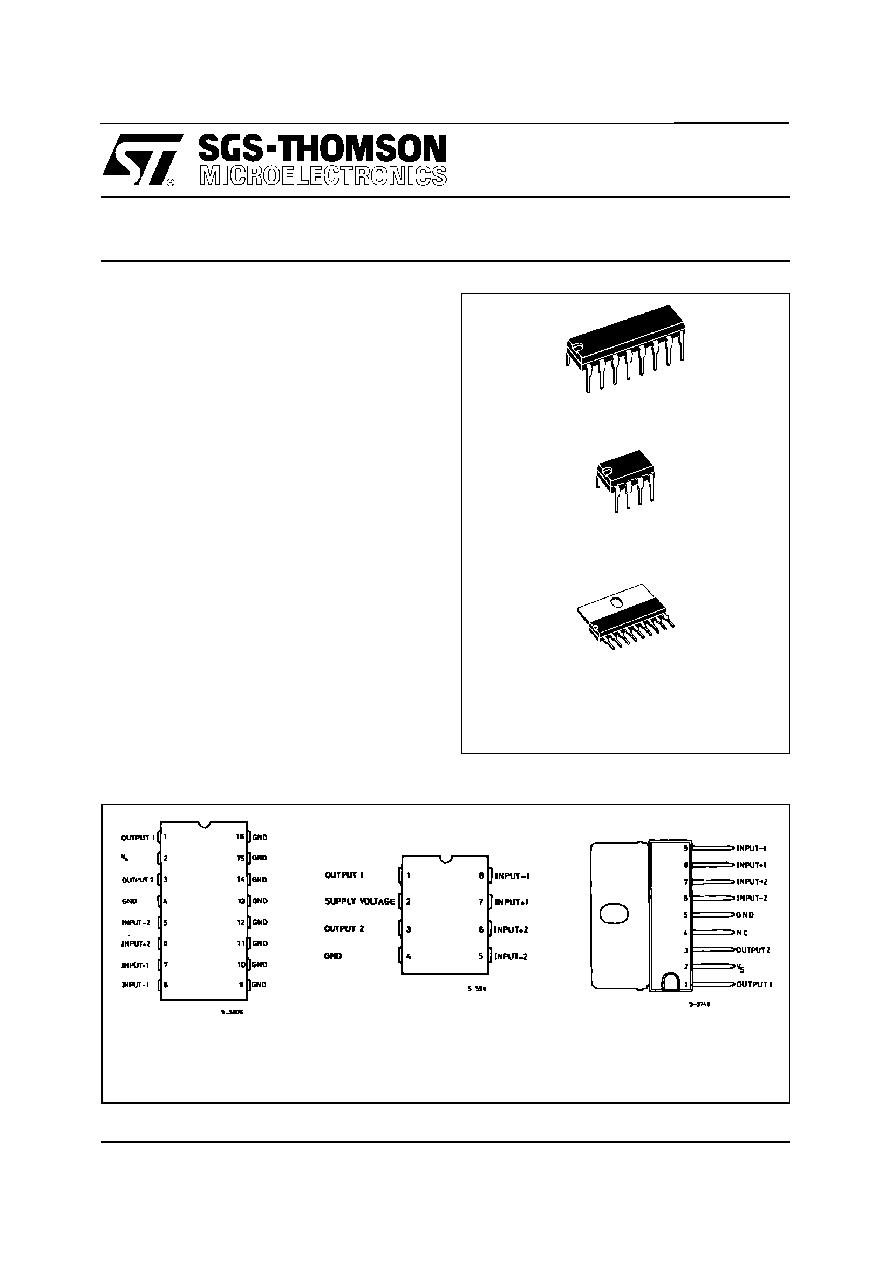L2720/2/4
November 1996
LOW DROP DUAL POWER OPERATIONAL AMPLIFIERS
.
OUTPUT CURRENT TO 1 A
.
OPERATES AT LOW VOLTAGES
.
SINGLE OR SPLIT SUPPLY
.
LARGE COMMON-MODE AND DIFFEREN-
TIAL MODE RANGE
.
LOW INPUT OFFSET VOLTAGE
.
GROUND COMPATIBLE INPUTS
.
LOW SATURATION VOLTAGE
.
THERMAL SHUTDOWN
.
CLAMP DIODE
DESCRIPTION
The L2720, L2722 and L2724 are monolithic inte-
grated circuits in powerdip, minidip and SIP-9 pack-
ages, intended for use as power operational ampli-
fiers in a wide range of applications including servo
amplifiers and power supplies.
They are particularly indicated for driving, inductive
loads, as motor and finds applications in compact-
disc VCR automotive, etc.
The high gain and high output power capability pro-
vide superior performance whatever an operational
amplifier/power booster combination is required.
PO WERDI P
(8 + 8)
MINIDI P
(Plastic)
SI P9
ORDERING NUMBERS :
L2720 (Powerdip)
L2722 (Minidip)
L2724 (SIP9)
1/10
PIN CONNECTIONS (top views)
L2720
L2722
L2724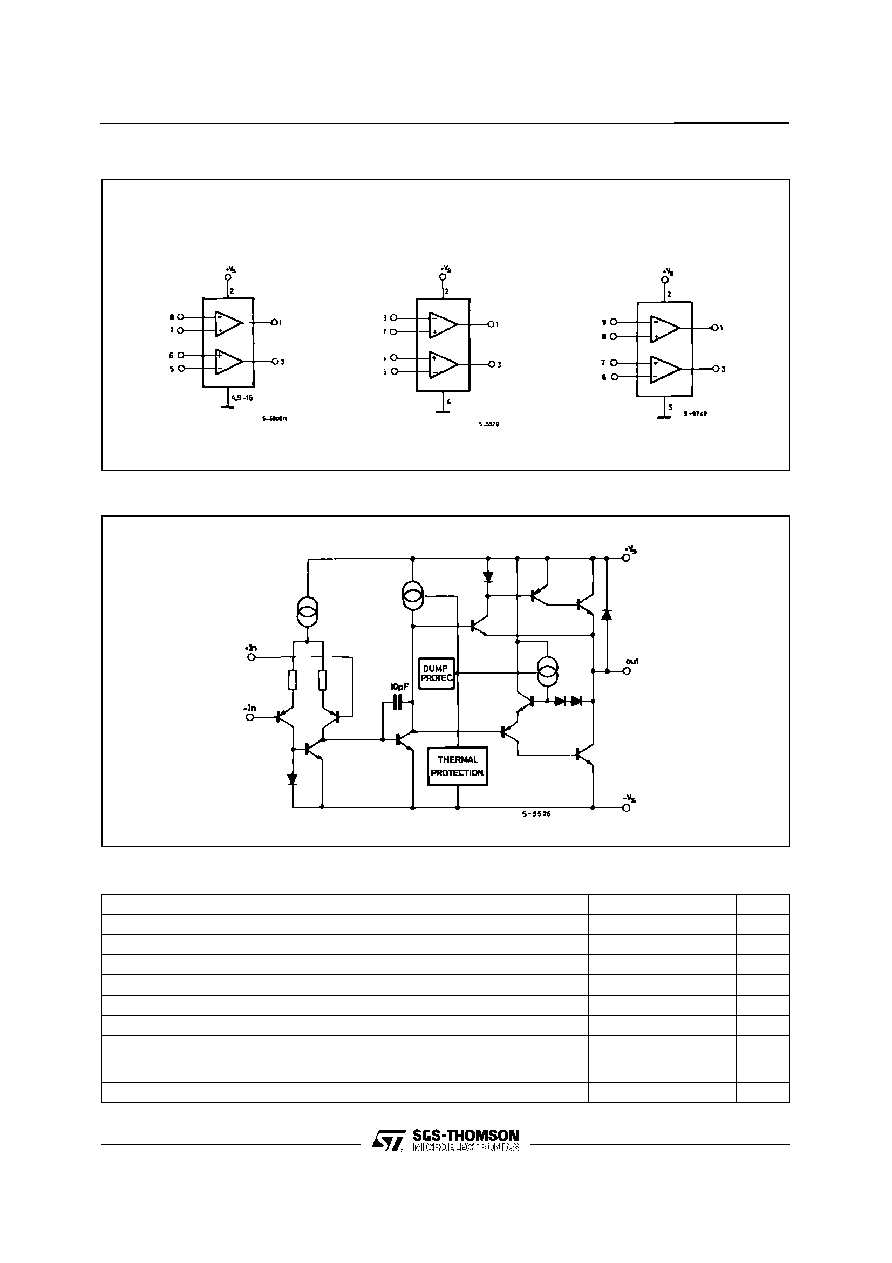BLOCK DIAGRAM
L2720
L2722
L2724
SCHEMATIC DIAGRAM (one section)
ABSOLUTE MAXIMUM RATINGS
Symbol
Parameter
Value
Unit
V
S
Supply Voltage
28
V
V
S
Peak Supply Voltage (50ms)
50
V
V
i
Input Voltage
V
s
V
i
Differential Input Voltage
±
V
s
I
o
DC Output Current
1
A
I
p
Peak Output Current (non repetitive)
1.5
A
P
tot
Power Dissipation at T
amb
= 80
o
C (L2720), T
amb
= 50
o
C (L2722)
T
case
= 75
o
C (L2720)
T
case
= 50
o
C (L2724)
1
5
10
W
T
stg
, T
j
Storage and Junction Temperature
­40 to 150
o
C
L2720/2/4
2/10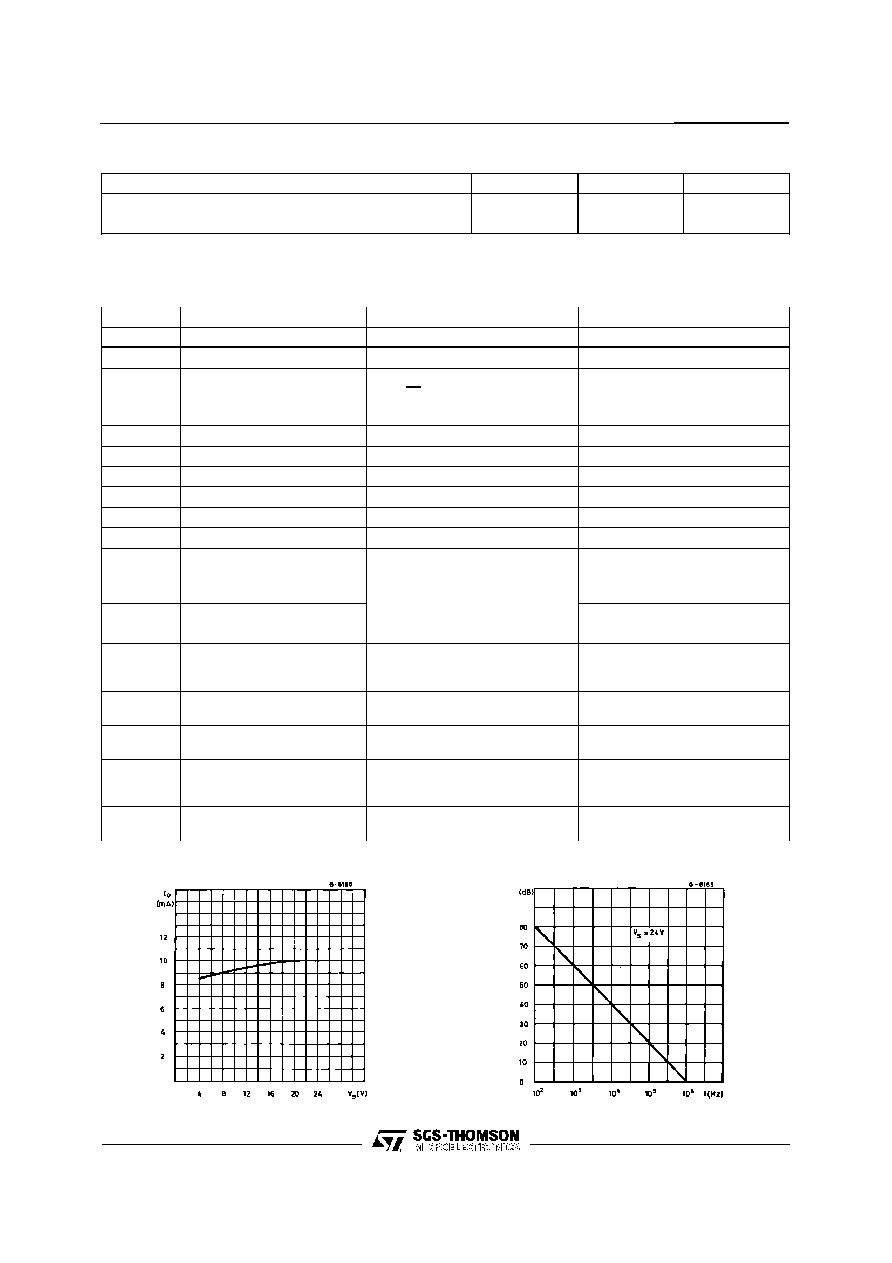THERMAL DATA
SIP-9
Powerdip
Minidip
R
th j-case
Thermal Resistance Junction-case
Max.
10
o
C/W
15
o
C/W
70
o
C/W
R
th j-amb
Thermal Resistance Junction-ambient
Max.
70
o
C/W
70
o
C/W
100
o
C/W
ELECTRICAL CHARACTERISTICS
V
s
= 24V, T
amb
= 25
o
C unless otherwise specified
Symbol
Parameter
Test Conditions
Min.
Typ.
Max.
Unit
V
s
Single Supply Voltage
4
28
V
V
s
Split Supply Voltage
±
2
±
14
V
I
s
Quiescent Drain Current
V
o
=
V
s
2
V
s
= 24V
10
15
mA
V
s
= 8V
9
15
I
b
Input Bias Current
0.2
1
µ
A
V
os
Input Offset Voltage
10
mV
I
os
Input Offset Current
100
nA
SR
Slew Rate
2
V/
µ
s
B
Gain-bandwidth Product
1.2
MHz
R
i
Input Resistance
500
k
G
v
O.L. Voltage Gain
f = 100Hz
f = 1kHz
70
80
60
dB
e
N
Input Noise Voltage
B = 22Hz to 22kHz
10
µ
V
I
N
Input Noise Voltage
200
pA
CMR
Common Mode Rejection
f = 1kHz
66
84
dB
SVR
Supply Voltage Rejection
f = 100Hz
V
s
= 24V
R
G
= 10k
V
s
=
±
12V
V
R
= 0.5V
V
s
=
±
6V
60
70
75
80
dB
V
DROP(HIGH)
V
s
=
±
2.5V to
±
12V
I
p
= 100mA
I
p
= 500mA
0.7
1
1.5
V
V
DROP(LOW)
V
s
=
±
2.5V to
±
12V
I
p
= 100mA
I
p
= 500mA
0.3
0.5
1
V
C
s
Channel Separation
f = 1KHz
V
s
= 24V
R
L
= 10
V
s
= 6V
G
v
= 30dB
60
60
dB
T
sd
Thermal Shutdown Junction
Temperature
145
o
C
Figure 1 :
Quiescent Current vs. Supply Voltage
FIgure 2 :
Open Loop Gain vs. Frequency
L2720/2/4
3/10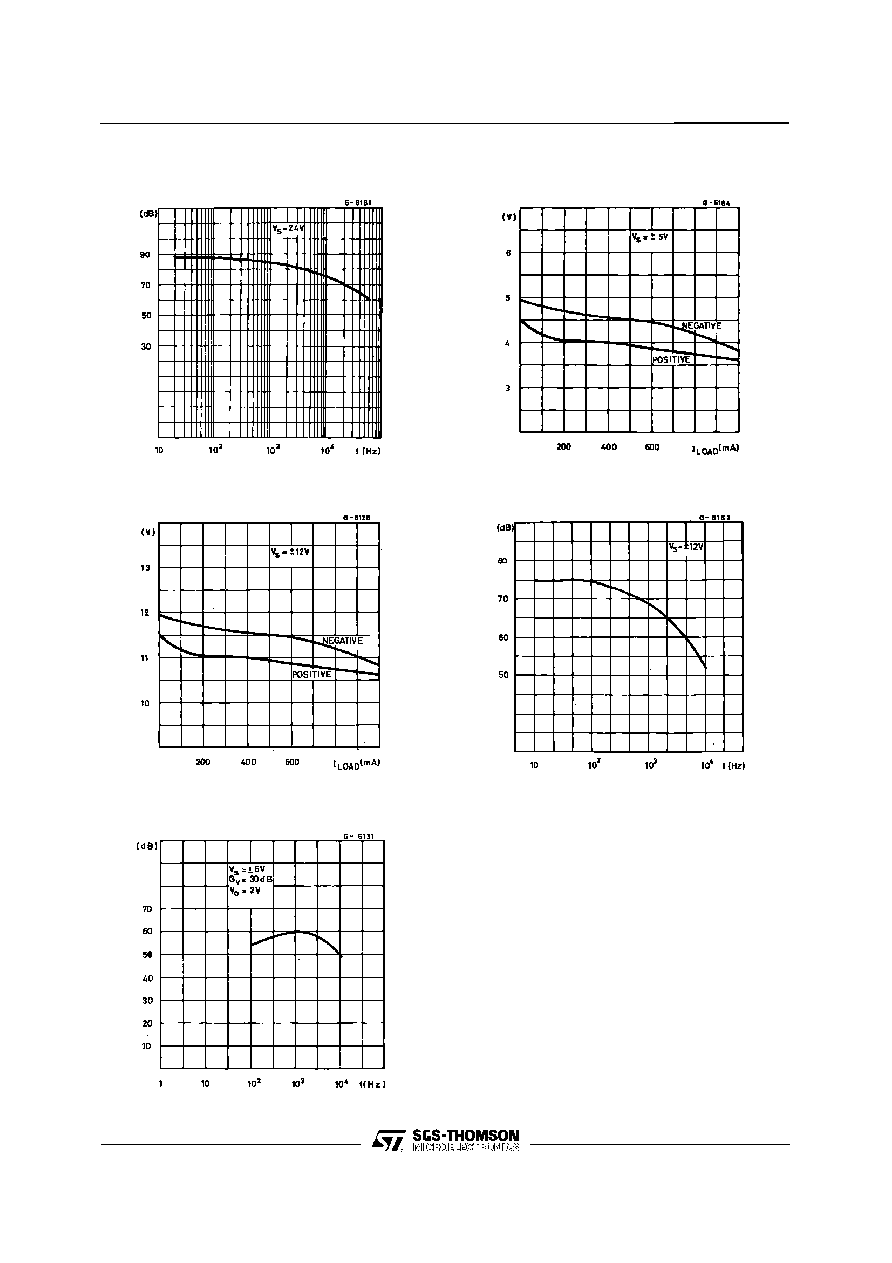Figure 3 :
Common Mode Rejection vs.
Frequency
Figure 4 :
Output Swing vs. Load Current
(V
S
=
±
5 V.
Figure 5 :
Output Swing vs. Load Current
(V
S
=
±
12 V.
Figure 6 :
Supply Voltage rejection vs.
Frequency
Figure 7 :
Channel Separation vs.
Frequency
L2720/2/4
4/10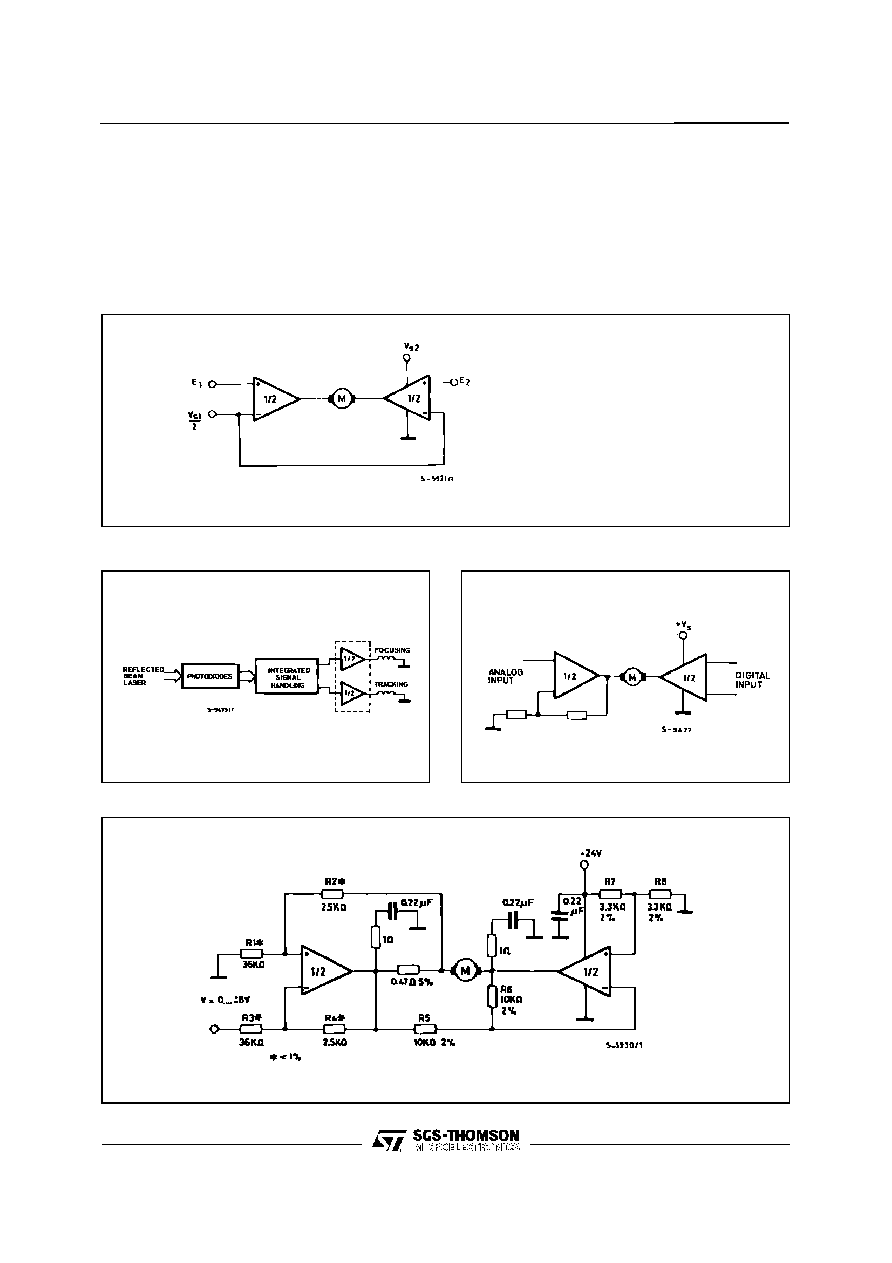APPLICATION SUGGESTION
In order to avoid possible instability occuring into fi-
nal stage the usual suggestions for the linear power
stages are useful, as for instance :
-
layout accuracy ;
-
A 100nFcapacitor connectedbetween supply
pins and ground ;
-
boucherot cell (0.1 to 0.2
µ
F + 1
series) be-
tween outputs and ground or across the load.
With single supply operation, a resistor (1k
)
between the output and supply pin can be
necessary for stability.
Figure 8 :
Bidirectional DC Motor Control with
µ
P Compatible Inputs
V
S1
= logic supply voltage
Must be V
S2
> V
S1
E1, E2 = logic inputs
Figure 9 :
Servocontrol for Compact-disc
Figure 10 : Capstan Motor Control in Video
Recorders
Figure 11 : Motor Current Control Circuit
No te :
The input voltage level is compatible with L291 (8 - BIT D/A converter)
L2720/2/4
5/10© 2019 • ICSheet• Contact form• Main page